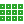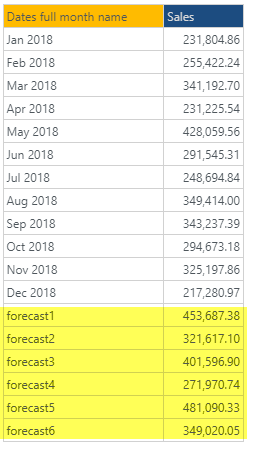#Forecast (PQL)

Returns a list of forecasted values for the number of periods specified using 'best fit'

• Returned Output: Numeric List
• Library: PQL \ Dynamic \ Grid
• Version: 2020.02.000
• Compatibility: Any content (regardless of data source) in the relevant parts of the application

#### Syntax

Forecast(, )

* Click on the function's arguments above for more details on the input values.

• The numeric listing is the set of 'existing' values that will drive the forecast formula.
• The forecast periods is the number of periods to forecast into the future,
• Forecasting uses the internal 'best fit' engine that appraises several forecasting algorithms and chooses the one with the highest statistical fit.

#### Examples

The example below uses the following example grid.

Note that the yellow forecasted results are shown to demonstrate the outcomes.This example returns a list of 6 numbers representing the future forecasted values based on the 2018 data: 453k,321k,401k etc.

Forecast(dataSetColumn(0), 6)